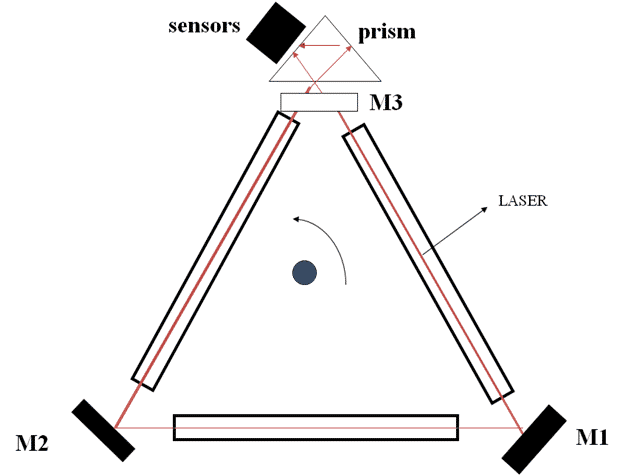Saturday, February 4, 2023

# Sagnac EffectSagnac effect : The Difference in path Length (∆L) is directional proportional to Angular Rotation (ω).

• Path traveled by F1=2πR
• Path traveled by F2=2πR

When Course changes:-

• Path traveled by F1= 2πR+AB
• Path traveled by F2= 2πR – AB

Difference in Path Length i.e.

∆L  =  2AB

or ∆L = 2RӨ          (Since AB=RӨ)

Or    ∆L = 2R ωT       (As D=S x T)

= 2Rω x 2πR    (Where 2πR is Total Distance)
= 4πR² ω/C (C=Speed of Light)

Therefore,  ∆L = K ω( As Area of Circle 4πR² & Speed Of light C are
Constants)

Proved: “∆L αω Difference In path Length is directly proportional to Angular Rotation.

Reference:

1.  F1 & F2 : Two similar Light Beams Traveling in Opposite Direction from ‘A’
2. If the apparatus is stationary the beams will meet each other at ‘A’. The path lengths of both beams will be same.
3. This results constructive interference of two beams.
4. If the apparatus rotates the beams will meet each other at ‘B’ and there will be a difference in the path lengths of two beams, F1 travels an additional distance of ‘AB’. Similarly, F2 travels a less distance of ‘AB’.
5. This results in net phase difference and destructive interference of two beams.
6. The net signal will vary in amplitude depending on the phase shift, therefore the resulting amplitude is a measurement of the phase shift, and consequently, the rotation rate.The same effect can be observed on Earth by sending radio signals through satellites around Earth in directions with and against the Earth’s rotation.

Sagnac effect contradicts the original theories of Special Relativity, since the fundamental theory of the constancy of the speed of light is violated in rotating systems. The Sagnac effect is not relativistic in nature, because one needs only small rotational velocities to observe it. When the perimeter velocities become large, the situation changes somewhat due to time dilation and Lorentz contraction, but the general result is the same.

Previous article
Next articleSahil Mishra
Sahil is a BTech in Marine Electrical. He is also a Reliability Analyst and has specializations in Russian Control Systems, Machinery Control System and Automated Power Management. He has work experience of 18 years. He is an Author, Editor and Partner at Electricalfundablog.
RELATED ARTICLES